# Classical mechanics facts for kids

Kids Encyclopedia Facts
(Redirected from Newtonian physics)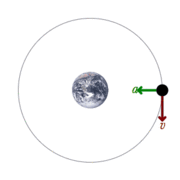Diagram of orbital motion of a satellite around the earth, showing perpendicular velocity and acceleration (force) vectors.

In physics, classical mechanics is one of two major sub-fields of mechanics. The other sub-field is quantum mechanics. Classical mechanics is concerned with the set of physical laws describing the motion of bodies under the influence of a system of forces. The study of the motion of bodies is an ancient one, making classical mechanics one of the oldest and largest subjects in science, engineering and technology. It is also known as Newtonian mechanics, though textbook authors often consider Newtonian mechanics, along with Lagrangian mechanics and Hamiltonian mechanics, as the three main formalisms of classical mechanics.

Classical mechanics describes the motion of macroscopic objects, from projectiles to parts of machinery, and astronomical objects, such as spacecraft, planets, stars and galaxies. Within classical mechanics are sub-fields, including those that describe the behavior of solids, liquids and gases. Classical mechanics provides extremely accurate results when studying large objects and speeds not approaching the speed of light.

When the objects being examined are sufficiently small, it becomes necessary to introduce the other major sub-field of mechanics: quantum mechanics. This sub-field adjusts the laws of physics of macroscopic objects for the atomic nature of matter by including the wave–particle duality of atoms and molecules.

When neither quantum nor classical mechanics apply, such as at the quantum level with high speeds, quantum field theory (QFT) becomes applicable.

The term classical mechanics was coined in the early 20th century. It describes the system of physics started by Isaac Newton and many contemporary 17th century natural philosophers. It is also built upon the earlier astronomical theories of Johannes Kepler. Classical mechanics uses common-sense notions of how matter and forces exist and interact. It assumes that matter and energy have definite, knowable attributes such as location in space and speed.

## Newton's Three Laws

Newton's three laws of motion are important to classical mechanics. Isaac Newton made them.

The first law says that, if there is no external force (meaning there is no pushing, gravity, or any sort of power), things that are stopped will stay stopped or un-moving, and things that are moving will keep moving. Before, people thought that things stopped if there was no force present. Often, people say, Objects that are stopped tend to stay stopped, and objects that are moving tend to stay moving, unless acted upon by an outside force, such as gravity, friction, etc....

The second law says how a force moves a thing. The net force on an object equals the rate of change of its momentum.

The third law says that if one thing puts a force on another thing, the second thing also puts a force on the first thing. The second force is equal in size to the first force. The forces act in opposite directions. For example, if you jump forward off a boat, the boat moves backward. Often, people say, For every action there is an equal and opposite reaction.

## Kinematic Equations

In physics, kinematics is the part of classical mechanics that explains the movement of objects without looking at what causes the movement or what the movement affects.

### 1-Dimensional Kinematics

1-Dimensional (1D) Kinematics are used only when an object moves in one direction: either side to side (left to right) or up and down. There are equations with can be used to solve problems that have movement in only 1 dimension or direction. These equations come from the definitions of velocity, acceleration and distance.

1. The first 1D kinematic equation deals with acceleration and velocity. If acceleration and velocity do not change. (Does not need include distance)
Equation: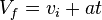$V_f=v_i+at$
Vf is the final velocity.
vi is the starting or initial velocity
a is the acceleration
t is time - how long the object was accelerated for.
2. The second 1D kinematic equation finds the distance moved, by using the average velocity and the time. (Does not need include acceleration)
Equation: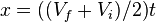$x=((V_f+V_i)/2)t$
x is the distance moved.
Vf is the final velocity.
vi is the starting or initial velocity
t is time
3. The third 1D kinematic equation finds the distance travelled, while the object is accelerating. It deals with velocity, acceleration, time and distance. (Does not need include final velocity)
Equation:$X_f=x_i+v_it+(1/2)at^2$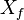$X_f$ is the final distance moved
xi is the starting or initial distance
vi is the starting or initial velocity
a is the acceleration
t is time
4. The fourth 1D kinematic equation finds the final velocity by using the initial velocity, acceleration and distance travelled. (Does not need include time)
Equation: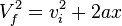$V_f^2=v_i^2+2ax$
Vf is the final velocity
vi is the starting or initial velocity
a is the acceleration
x is the distance moved

### 2-Dimensional Kinematics

2-Dimensional kinematics is used when motion happens in both the x-direction (left to right) and the y-direction (up and down). There are also equations for this type of kinematics. However, there are different equations for the x-direction and different equations for the y-direction. Galileo proved that the velocity in the x-direction does not change through the whole run. However, the y-direction is affected by the force of gravity, so the y-velocity does change during the run.

#### X-Direction Equations

Left and Right movement
1. The first x-direction equation is the only one that is needed to solve problems, because the velocity in the x-direction stays the same.
Equation: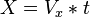$X=V_x*t$
X is the distance moved in the x-direction
Vx is the velocity in the x-direction
t is time

#### Y-Direction Equations

Up and Down movement. Affected by gravity or other external acceleration
1. The first y-direction equation is almost the same as the first 1-Dimensional kinematic equation except it deals with the changing y-velocity. It deals with a freely falling body while its being affected by gravity. (Distance is not needed)
Equation: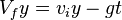$V_fy=v_iy-gt$
Vfy is the final y-velocity
viy is the starting or initial y-velocity
g is the acceleration because of gravity which is 9.8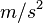$m/s^2$ or 32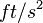$ft/s^2$
t is time
2. The second y-direction equation is used when the object is being affected by a separate acceleration, not by gravity. In this case, the y-component of the acceleration vector is needed. (Distance is not needed)
Equation: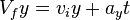$V_fy=v_iy+a_yt$
Vfy is the final y-velocity
viy is the starting or initial y-velocity
ay is the y-component of the acceleration vector
t is the time
3. The third y-direction equation finds the distance moved in the y-direction by using the average y-velocity and the time. (Does not need acceleration of gravity or external acceration)
Equation: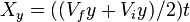$X_y=((V_fy+V_iy)/2)t$
Xy is the distance moved in the y-direction
Vfy is the final y-velocity
viy is the starting or initial y-velocity
t is the time
4. The fourth y-direction equation deals with the distance moved in the y-direction while being affected by gravity. (Does not need final y-velocity)
Equation: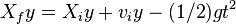$X_fy=X_iy+v_iy-(1/2)gt^2$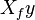$X_fy$ is the final distance moved in the y-direction
xiy is the starting or initial distance in the y-direction
viy is the starting or initial velocity in the y-direction
g is the acceleration of gravity which is 9.8$m/s^2$ or 32$ft/s^2$
t is time
5. The fifth y-direction equation deals with the distance moved in the y-direction while being affected by a different acceleration other than gravity. (Does not need final y-velocity)
Equation: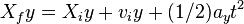$X_fy=X_iy+v_iy+(1/2)a_yt^2$$X_fy$ is the final distance moved in the y-direction
xiy is the starting or initial distance in the y-direction
viy is the starting or initial velocity in the y-direction
ay is the y-component of the acceleration vector
t is time
6. The sixth y-direction equation finds the final y-velocity while it is being affected by gravity over a certain distance. (Does not need time)
Equation: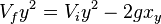$V_fy^2=V_iy^2-2gx_y$
Vfy is the final velocity in the y-direction
Viy is the starting or initial velocity in the y-direction
g is the acceleration of gravity which is 9.8$m/s^2$ or 32$ft/s^2$
xy is the total distance moved in the y-direction
7. The seventh y-direction equation finds the final y-velocity while it is being affected by an acceleration other than gravity over a certain distance. (Does not need time)
Equation: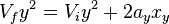$V_fy^2=V_iy^2+2a_yx_y$
Vfy is the final velocity in the y-direction
Viy is the starting or initial velocity in the y-direction
ay is the y-component of the acceleration vector
xy is the total distance moved in the y-direction

## Branches

Classical mechanics was traditionally divided into three main branches:

• Statics, the study of equilibrium and its relation to forces
• Dynamics, the study of motion and its relation to forces
• Kinematics, dealing with the implications of observed motions without regard for circumstances causing them

Another division is based on the choice of mathematical formalism:

Alternatively, a division can be made by region of application:

• Celestial mechanics, relating to stars, planets and other celestial bodies
• Continuum mechanics, for materials modelled as a continuum, e.g., solids and fluids (i.e., liquids and gases).
• Relativistic mechanics (i.e. including the special and general theories of relativity), for bodies whose speed is close to the speed of light.
• Statistical mechanics, which provides a framework for relating the microscopic properties of individual atoms and molecules to the macroscopic or bulk thermodynamic properties of materials.

## Images for kidsClassical mechanics Facts for Kids. Kiddle Encyclopedia.